#### The Data Science Lab### Anomaly Detection Using Principal Component Analysis (PCA)

The main advantage of using PCA for anomaly detection, compared to alternative techniques such as a neural autoencoder, is simplicity -- assuming you have a function that computes eigenvalues and eigenvectors.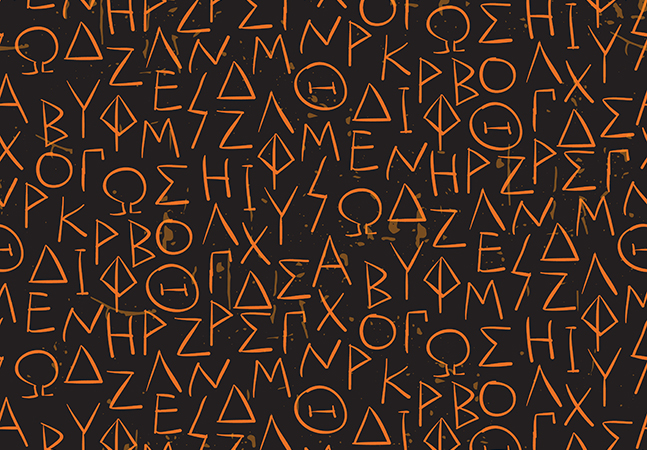### Ordinal Classification Using PyTorch

Dr. James McCaffrey of Microsoft Research presents a simple technique he has used with good success, previously unpublished and without a standard name.### Computing the Similarity Between Two Machine Learning Datasets

At first thought, computing the similarity/distance between two datasets sounds easy, but in fact the problem is extremely difficult, explains Dr. James McCaffrey of Microsoft Research.### Differential Evolution Optimization

Dr. James McCaffrey of Microsoft Research explains stochastic gradient descent (SGD) neural network training, specifically implementing a bio-inspired optimization technique called differential evolution optimization (DEO).### Wasserstein Distance Using C# and Python

Dr. James McCaffrey of Microsoft Research shows how to compute the Wasserstein distance and explains why it is often preferable to alternative distance functions, used to measure the distance between two probability distributions in machine learning projects.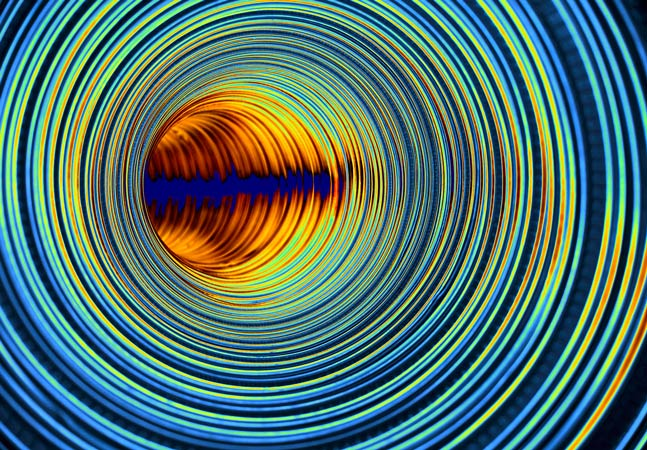### Spiral Dynamics Optimization with Python

Dr. James McCaffrey of Microsoft Research explains how to implement a geometry-inspired optimization technique called spiral dynamics optimization (SDO), an alternative to Calculus-based techniques that may reach their limits with huge neural networks.### Sentiment Analysis Using a PyTorch EmbeddingBag Layer

Dr. James McCaffrey of Microsoft Research uses a full movie review example to explain the natural language processing (NLP) problem of sentiment analysis, used to predict whether some text is positive (class 1) or negative (class 0).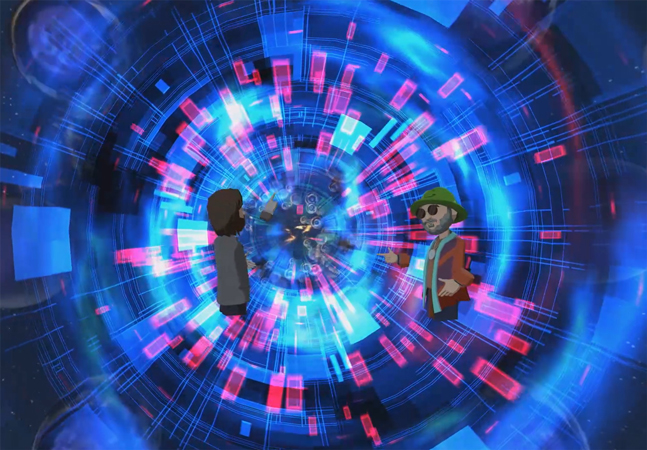### Logistic Regression Using PyTorch with L-BFGS

Dr. James McCaffrey of Microsoft Research demonstrates applying the L-BFGS optimization algorithm to the ML logistic regression technique for binary classification -- predicting one of two possible discrete values.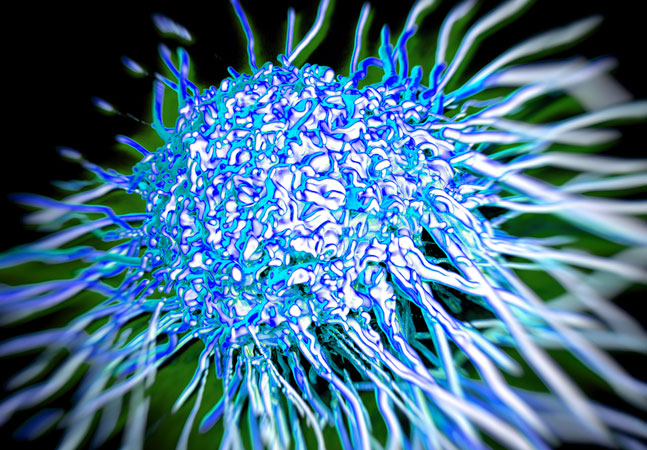### Generating Synthetic Data Using a Generative Adversarial Network (GAN) with PyTorch

Dr. James McCaffrey of Microsoft Research explains a generative adversarial network, a deep neural system that can be used to generate synthetic data for machine learning scenarios, such as generating synthetic males for a dataset that has many females but few males.### Positive and Unlabeled Learning (PUL) Using PyTorch

Dr. James McCaffrey of Microsoft Research provides a code-driven tutorial on PUL problems, which often occur with security or medical data in cases like training a machine learning model to predict if a hospital patient has a disease or not.### Generating Synthetic Data Using a Variational Autoencoder with PyTorch

Generating synthetic data is useful when you have imbalanced training data for a particular class, for example, generating synthetic females in a dataset of employees that has many males but few females.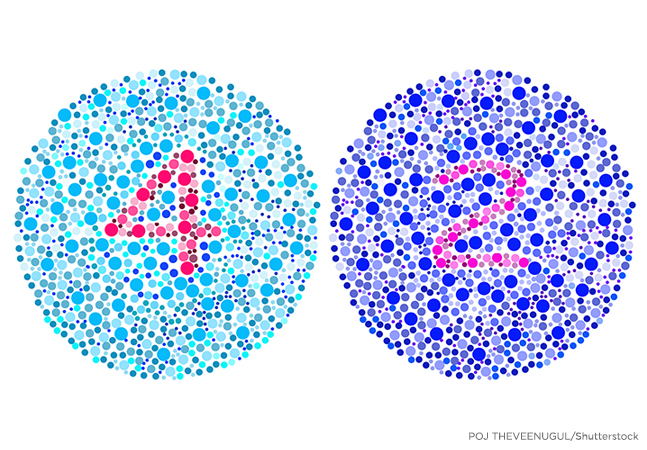### Autoencoder Anomaly Detection Using PyTorch

Dr. James McCaffrey of Microsoft Research provides full code and step-by-step examples of anomaly detection, used to find items in a dataset that are different from the majority for tasks like detecting credit card fraud.### How To: Create a Streaming Data Loader for PyTorch

When training data won't fit into machine memory, a streaming data loader using an internal memory buffer can help. Dr. James McCaffrey of Microsoft Research shows how.### Neural Regression Using PyTorch: Model Accuracy

Dr. James McCaffrey of Microsoft Research explains how to evaluate, save and use a trained regression model, used to predict a single numeric value such as the annual revenue of a new restaurant based on variables such as menu prices, number of tables, location and so on.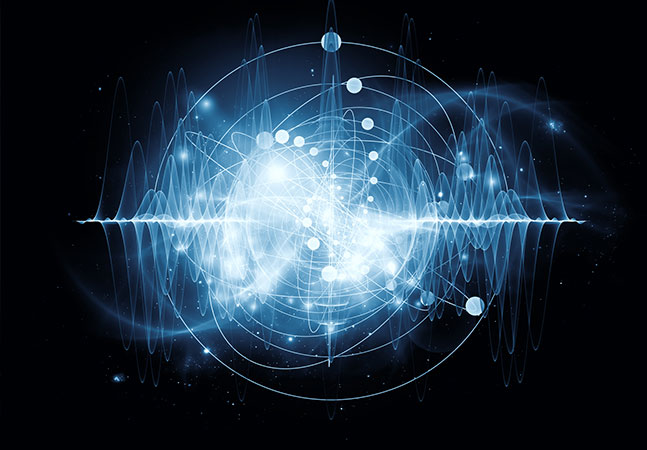### Neural Regression Using PyTorch: Training

The goal of a regression problem is to predict a single numeric value, for example, predicting the annual revenue of a new restaurant based on variables such as menu prices, number of tables, location and so on.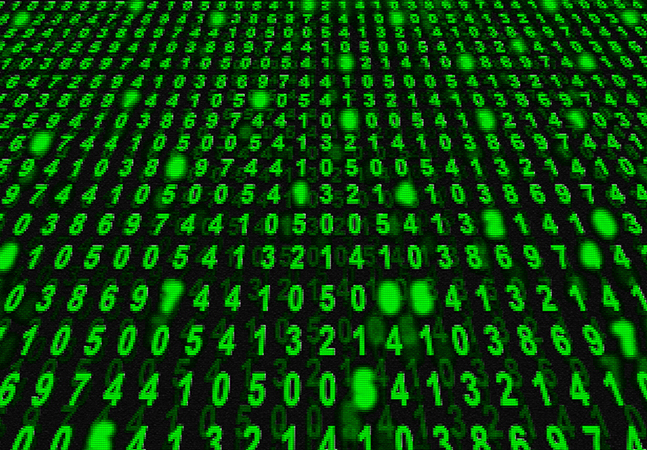### Neural Regression Using PyTorch: Defining a Network

Dr. James McCaffrey of Microsoft Research presents the second of four machine learning articles that detail a complete end-to-end production-quality example of neural regression using PyTorch.### Neural Regression Classification Using PyTorch: Preparing Data

Dr. James McCaffrey of Microsoft Research presents the first in a series of four machine learning articles that detail a complete end-to-end production-quality example of neural regression using PyTorch.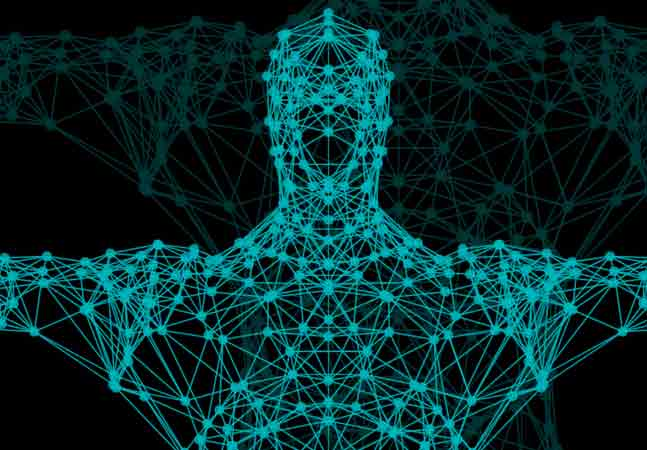### Multi-Class Classification Using PyTorch: Model Accuracy

Dr. James McCaffrey of Microsoft Research continues his four-part series on multi-class classification, designed to predict a value that can be one of three or more possible discrete values, by explaining model accuracy.### Multi-Class Classification Using PyTorch: Training

Dr. James McCaffrey of Microsoft Research continues his four-part series on multi-class classification, designed to predict a value that can be one of three or more possible discrete values, by explaining neural network training.### Multi-Class Classification Using PyTorch: Defining a Network

Dr. James McCaffrey of Microsoft Research explains how to define a network in installment No. 2 of his four-part series that will present a complete end-to-end production-quality example of multi-class classification using a PyTorch neural network.### Multi-Class Classification Using PyTorch: Preparing Data

Dr. James McCaffrey of Microsoft Research kicks off a four-part series on multi-class classification, designed to predict a value that can be one of three or more possible discrete values.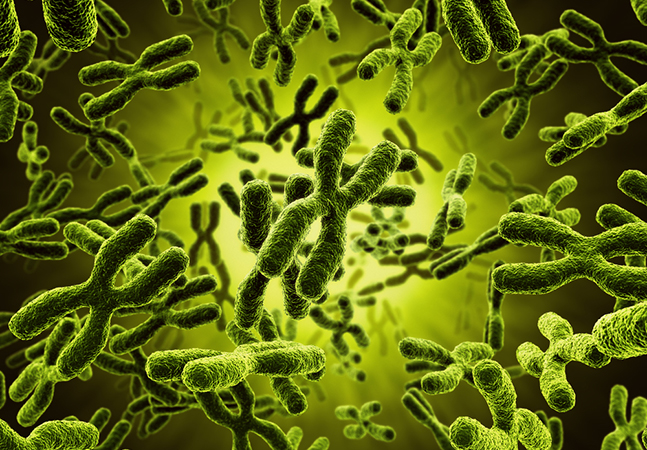### Binary Classification Using PyTorch: Model Accuracy

In the final article of a four-part series on binary classification using PyTorch, Dr. James McCaffrey of Microsoft Research shows how to evaluate the accuracy of a trained model, save a model to file, and use a model to make predictions.### Binary Classification Using PyTorch: Training

Dr. James McCaffrey of Microsoft Research continues his examination of creating a PyTorch neural network binary classifier through six steps, here addressing step No. 4: training the network.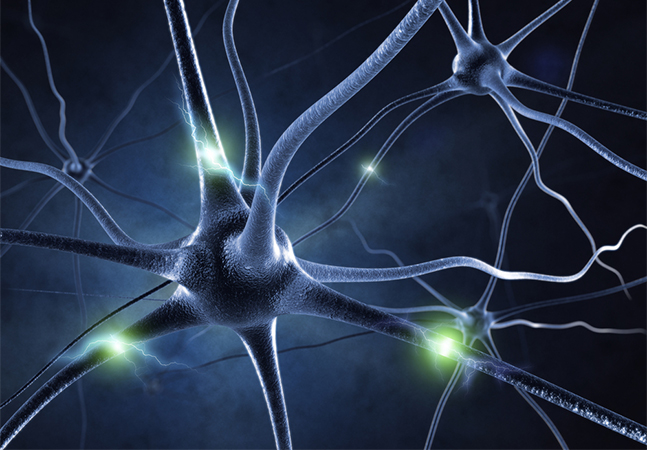### Binary Classification Using PyTorch: Defining a Network

Dr. James McCaffrey of Microsoft Research tackles how to define a network in the second of a series of four articles that present a complete end-to-end production-quality example of binary classification using a PyTorch neural network, including a full Python code sample and data files.### Binary Classification Using PyTorch: Preparing Data

Dr. James McCaffrey of Microsoft Research kicks off a series of four articles that present a complete end-to-end production-quality example of binary classification using a PyTorch neural network, including a full Python code sample and data files.

Upcoming Events